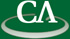## On the bit complexity of sparse integer polynomial interpolation.

### 10:30am Tuesday February 17th, 2015, in K9509.

```Abstract

We consider the multiplication of polynomials with integer coefficients,
given by sparse representations.  For this problem the number of terms in
the output can greatly vary from quadratic to constant with respect to the
number of terms in the inputs, depending on the cancellation of terms.  In
the event that the output has very few terms, grade-school multiplication
may have cost potentially quadratic with respect to the total size of the
inputs and output.  We present a probabilistic multiplication algorithm for
sparse polynomials that, assuming a naive height bound on the output, is
softly linear with respect to the combined bit size of the inputs and
output.  This algorithm is based off of techniques developed for sparse
interpolation of straight-line programs.

This is joint work with Dan Roche of the United States Naval Academy.

```